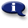# .

## Syllabus Information

Fall 2022
Jun 05,2023Use this page to maintain syllabus information, learning objectives, required materials, and technical requirements for the course.

Syllabus Information
MTH 243 - Introduction to Probability and Statistics
Associated Term: Fall 2022
Learning Objectives: Upon successful completion of this course, the student will be able to:
1. Classify data as categorical or quantitative
2. Construct frequency bar charts, histograms, boxplots, stemplots and scatterplots
3. Use descriptive statistics for center, location and spread
4. Demonstrate an understanding of probability experiments, outcomes and events
5. Recognize disjoint and independent events
6. Find and interpret theoretical and statistical probabilities, including compound and conditional probabilities
7. Find probabilities and values for a normal distribution
8. Find probabilities for uniform, binomial, and Poisson distributions
9. Use the Central Limit Theorem to construct confidence intervals for population mean
10. Construct confidence intervals for population proportion
11. Test hypotheses about one or two population means
12. Test hypotheses about one or two population proportions
13. Interpret the correlation coefficient and its square
14. Create regression lines and test their statistical significance
15. Test the independence of two categorical variables using Chi-square
16. Demonstrate an understanding of sampling and basic research design
17. Demonstrate an understanding of sources of bias and error in statistical research
18. Use statistical software and the statistical features of a graphing calculator
Required Materials:
Technical Requirements: